# RD Sharma solutions for Class 10 Maths chapter 13 - Areas Related to Circles [Latest edition]

#### Chapters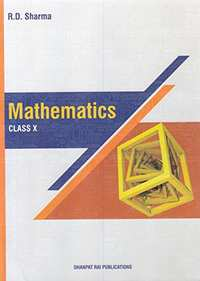## Chapter 13: Areas Related to Circles

Exercise 13.1Exercise 13.2Exercise 13.3Exercise 13.4Exercise 13.5Exercise 13.6
Exercise 13.1 [Pages 12 - 13]

### RD Sharma solutions for Class 10 Maths Chapter 13 Areas Related to Circles Exercise 13.1 [Pages 12 - 13]

Exercise 13.1 | Q 1 | Page 12

Find the circumference and area of circle of radius 4.2 cm

Exercise 13.1 | Q 2 | Page 12

Find the circumference of a circle whose area is 301.84 cm2.

Exercise 13.1 | Q 3 | Page 12

Find the area of circle whose circumference is 44 cm.

Exercise 13.1 | Q 4 | Page 12

The circumference of a circle exceeds diameter by 16.8 cm. Find the circumference of
circle.

Exercise 13.1 | Q 5 | Page 12

A horse is tied to a pole with 28m long string. Find the area where the horse can graze.

Exercise 13.1 | Q 6 | Page 12

A steel wire when bent is the form of square encloses an area of 12 cm2. If the same wire is bent in form of circle. Find the area of circle.

Exercise 13.1 | Q 7 | Page 12

A horse is placed for grazing inside a rectangular field 40m by 36m and is tethered to one corner by a rope 14m long. Over how much area can it graze.

Exercise 13.1 | Q 8 | Page 12

A sheet of paper is in the form of rectangle ABCD in which AB = 40cm and AD = 28 cm. A semicircular portion with BC as diameter is cut off. Find the area of remaining paper.

Exercise 13.1 | Q 9 | Page 12

The circumference of two circles are in ratio 2:3. Find the ratio of their areas

Exercise 13.1 | Q 10 | Page 12

The side of a square is 10 cm. find the area of circumscribed and inscribed circles.

Exercise 13.1 | Q 11 | Page 12

The sum of the radii of two circles is 140 cm and the difference of their circumferences in 88 cm. Find the diameters of the circles.

Exercise 13.1 | Q 12 | Page 12

The area of circle, inscribed in equilateral triangle is 154 cms2. Find the perimeter of
triangle.

Exercise 13.1 | Q 13 | Page 12

A field is in the form of circle. A fence is to be erected around the field. The cost of fencing would to Rs. 2640 at rate of Rs.12 per metre. Then the field is to be thoroughs ploughed at cost of Rs. 0.50 per m2. What is amount required to plough the field?

Exercise 13.1 | Q 14 | Page 12

If a square is inscribed in a circle, find the ratio of areas of the circle and the square.

Exercise 13.1 | Q 15 | Page 12

A park is in the form of rectangle 120m × 100m. At the centre of park there is a circular lawn. The area of park excluding lawn is 8700m2. Find the radius of circular lawn.

Exercise 13.1 | Q 16 | Page 12

The radii of two circles are 8 cm and 6 cm respectively. Find the radius of the circle having area equal to the sum of the areas of the two circles.

Exercise 13.1 | Q 17 | Page 12

The radii of two circles are 19cm and 9 cm respectively. Find the radius and area of the circle which has circumferences is equal to sum of circumference of two circles.

Exercise 13.1 | Q 18 | Page 12

A car travels 1 km distance in which each wheel makes 450 complete revolutions. Find the radius of wheel.

Exercise 13.1 | Q 19 | Page 12

The area enclosed between the concentric circles is 770cm2. If the radius of outer circle 21cm. find the radius of inner circle

Exercise 13.1 | Q 20 | Page 12

An archery target has three regions formed by three concentric circles as shown in figure. If the diameters of the concentric circles are in the ratios 1 : 2 : 3 , then find the ratio of the areas of three regions.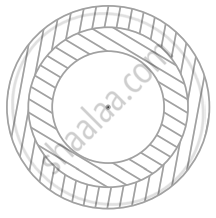Exercise 13.1 | Q 21 | Page 13

The wheel of a motor cycle is of radius 35 cm . How many revolutions per minute must the wheel make so as to keep a speed of 66 km / hr ?

Exercise 13.1 | Q 22 | Page 13

A circular pond is 17.5 m in diameter. It is surrounded by a 2m wide path, Find the cost of constructing the path at the rate of ₹ 25 per m.

Exercise 13.1 | Q 23 | Page 13

A circular park is surroundeed by a rod 21 m wide. If the radius of the park is 105 m, find the area of the road .

Exercise 13.1 | Q 24 | Page 13

A square of diagonal 8 cm is inscribed in a circle. Find the area of the region lying outside the circle and inside the square .

Exercise 13.1 | Q 26 | Page 13

Find the area enclosed between two concentric circles of radii 3.5 cm and 7 cm. A third concentric circle is drawn outside the 7 cm circle , such that the area enclosed between it and the 7 cm circle is same as that between the two inner circles . Find the radius of the third circle correct to one decimal place.

Exercise 13.1 | Q 27 | Page 13

A path of width 3.5 m  runs around a semi-circular grassy plot whose perimeter is 72 m . Find the area of the path .

("Use"  pi= 22/7)

Exercise 13.1 | Q 28 | Page 13

A circular pond is of diameter 17.5 m . It is surrounded by a 2m wide path . Find the cost of constructing the path at the rate of ₹25 per square metre (Use pi=22/7)

Exercise 13.1 | Q 29 | Page 13

The outer circumference of a circular race-track is 528 m . The track is everywhere 14 m wide. Calculate the cost of levelling the track at the rate of 50 paise per square metre.

(use pi=22/7).

Exercise 13.1 | Q 30 | Page 13

A road which is 7 m wide surrounds a circular park whose circumference  is 352 m . Find the area of the road .

Exercise 13.1 | Q 31 | Page 13

Prove that the area of a circular path of uniform width h surrounding a circular region of radius r is pih(2r+h)

Exercise 13.2 [Pages 24 - 26]

### RD Sharma solutions for Class 10 Maths Chapter 13 Areas Related to Circles Exercise 13.2 [Pages 24 - 26]

Exercise 13.2 | Q 1 | Page 24

Find in terms of x the length of the arc that subtends an angle of 30°, at the centre of circle of radius 4 cm.

Exercise 13.2 | Q 2 | Page 24

Find the angle subtended at the centre of circle of radius 5cm by an arc of length ((5pi)/3) cm

Exercise 13.2 | Q 3 | Page 24

An arc of length 20𝜋 cm subtends an angle of 144° at centre of circle. Find the radius of the circle.

Exercise 13.2 | Q 4 | Page 24

An arc of length 15 cm subtends an angle of 45° at the centre of a circle. Find in terms of 𝜋, radius of the circle.

Exercise 13.2 | Q 5 | Page 24

Find the angle subtended at the centre of circle of radius ‘a’ cm by an arc of length
(api)/4 𝑐𝑚

Exercise 13.2 | Q 6 | Page 24

A sector of circle of radius 4cm contains an angle of 30°. Find the area of sector

Exercise 13.2 | Q 7 | Page 24

A sector of a circle of radius 8cm contains the angle of 135°. Find the area of sector.

Exercise 13.2 | Q 8 | Page 25

The area of sector of circle of radius 2cm is 𝜋cm2. Find the angle contained by the sector.

Exercise 13.2 | Q 9 | Page 25

The area of sector of circle of radius 5cm is 5𝜋 cm2. Find the angle contained by the sector.

Exercise 13.2 | Q 10 | Page 25

AB is a chord of circle with centre O and radius 4cm. AB is length of 4cm. Find the area of sector of the circle formed by chord AB

Exercise 13.2 | Q 11 | Page 25

In a circle of radius 35 cm, an arc subtends an angle of 72° at the centre. Find the length of arc and area of sector

Exercise 13.2 | Q 12 | Page 25

The perimeter of a sector of circle of radius 5.7m is 27.2 m. Find the area of sector.

Exercise 13.2 | Q 13 | Page 25

The perimeter of certain sector of circle of radius 5.6 m is 27.2 m. Find the area of sector.

Exercise 13.2 | Q 14 | Page 25

A sector is cut-off from a circle of radius 21 cm the angle of sector is 120°. Find the length of its arc and its area.

Exercise 13.2 | Q 15 | Page 25

The minute hand of a clock is √21 𝑐𝑚 long. Find area described by the minute hand on the face of clock between 7 am and 7:05 am

Exercise 13.2 | Q 16 | Page 25

The minute hand of clock is10cm long. Find the area of the face of the clock described by the minute hand between 8am and 8:25 am

Exercise 13.2 | Q 17 | Page 25

A sector of 56° cut out from a circle contains area of 4.4 cm2. Find the radius of the circle

Exercise 13.2 | Q 18 | Page 25

Area of sector of central angle 2000 of  a circle  is 770 cm2 . Find the length of the corresponding arc of this sector .

Exercise 13.2 | Q 19 | Page 25

The length of minute hand of a clock is 5 cm . Find the area swept by the minute hand  during the time period 6:05 am and 6:40 am.

Exercise 13.2 | Q 20 | Page 25

The length of the minute hand of a clock is 14 cm. Find the area swept by the minute hand in 5 minutes.

Exercise 13.2 | Q 21.1 | Page 25

In a circle of radius 21 cm, an arc subtends an angle of 60° at the centre. Find the length of the arc  (Use π = 22/7)

Exercise 13.2 | Q 21.2 | Page 25

In a circle of radius 21 cm, an arc subtends an angle of 60° at the centre. Find area of the sector formed by the arc. (Use π = 22/7)

Exercise 13.2 | Q 22 | Page 25

From a circular piece of cardboard of radius 3 cm two sectors of 900 have been cutoff . Find the perimeter of the remaining portion nearest hundredth centimeters ( "Take"  pi = 22/ 7).

Exercise 13.2 | Q 23 | Page 25

The area of sector is one-twelfth that of the complete circle. Find the angle of the sector .

Exercise 13.2 | Q 24 | Page 25

AB is a chord of a circle with centre O and radius 4 cm. AB is of length 4 cm. Find the area of the sector of the circle formed by chord AB.

Exercise 13.2 | Q 25.1 | Page 25

In circle of radius 6cm, chord of length 10 cm makes an angle of 110° at the centre of circle find Circumference of the circle

Exercise 13.2 | Q 25.2 | Page 25

In circle of radius 6cm, chord of length 10 cm makes an angle of 110° at the centre of circle find Area of the circle

Exercise 13.2 | Q 25.3 | Page 25

In circle of radius 6cm, chord of length 10 cm makes an angle of 110° at the centre of circle find Length of arc

Exercise 13.2 | Q 25.4 | Page 25

In circle of radius 6cm, chord of length 10 cm makes an angle of 110° at the centre of circle find The area of sector

Exercise 13.2 | Q 26.1 | Page 25

Below fig shows a sector of a circle, centre O. containing an angle 𝜃°. Prove that

Area of shaded region isr^2/2(tantheta −(pitheta)/180)

Exercise 13.2 | Q 26.1 | Page 25

Below fig shows a sector of a circle, centre O. containing an angle 𝜃°. Prove that Perimeter of shaded region is 𝑟 (tan 𝜃 + sec 𝜃 +(pitheta)/180− 1)

Exercise 13.2 | Q 27 | Page 26

The diagram shows a sector of circle of radius ‘r’ can containing an angle 𝜃. The area of sector is A cm2 and perimeter of sector is 50 cm. Prove that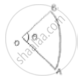(i) 𝜃 =360/pi(25/r− 1)

(ii) A = 25r – r2

Exercise 13.3 [Pages 32 - 33]

### RD Sharma solutions for Class 10 Maths Chapter 13 Areas Related to Circles Exercise 13.3 [Pages 32 - 33]

Exercise 13.3 | Q 1 | Page 32

AB is a chord of a circle with centre O and radius 4 cm. AB is of length 4 cm and divides the circle into two segments. Find the area of the minor segment.

Exercise 13.3 | Q 2 | Page 32

A chord PQ of length 12 cm subtends an angle of 120° at the centre of a circle. Find the area of the minor segment cut off by the chord PQ

Exercise 13.3 | Q 3 | Page 32

A chord of circle of radius 14cm makes a right angle at the centre. Find the areas of minor and major segments of the circle.

Exercise 13.3 | Q 4 | Page 32

A chord 10 cm long is drawn in a circle whose radius is 5√2 cm. Find the area of both
segments

Exercise 13.3 | Q 5 | Page 32

A chord AB of circle, of radius 14cm makes an angle of 60° at the centre. Find the area of minor segment of circle.

Exercise 13.3 | Q 6 | Page 32

Find the area of minor segment of a circle of radius 14 cm, when the angle of the corresponding sector is 600 .

Exercise 13.3 | Q 7 | Page 32

A chord of a circle of radius 20 cm sub tends an angle of 900 at the centre . Find the area of the corresponding major segment of the circle
( Use $\pi = 3 . 14$)

Exercise 13.3 | Q 9 | Page 33

AB is the diameter of a circle, centre O. C is a point on the circumference such that ∠COB = 𝜃. The area of the minor segment cutoff by AC is equal to twice the area of sector BOC.Prove that "sin"theta/2. "cos"theta/2= pi (1/2−theta/120^@)

Exercise 13.3 | Q 10 | Page 33

A chord of a circle subtends an angle 𝜃 at the centre of circle. The area of the minor segment cut off by the chord is one eighth of the area of circle. Prove that 8 sintheta/2 "cos"theta/2+pi =(pitheta)/45

Exercise 13.4 [Pages null - 65]

### RD Sharma solutions for Class 10 Maths Chapter 13 Areas Related to Circles Exercise 13.4 [Pages null - 65]

Exercise 13.4 | Q 1

A plot is in the form of rectangle ABCD having semi-circle on BC. If AB = 60m and BC = 28m, find the area of plot.

Exercise 13.4 | Q 2

A playground has the shape of rectangle, with two semicircles on its smaller sides as diameters, added to its outside. If the sides of rectangle are 36m and 24.5m. find the area of playground.

Exercise 13.4 | Q 3 | Page 56

Find the area of the circle in which a square of area 64 cm2 is inscribed. [Use π = 3.14]

Exercise 13.4 | Q 4

A rectangular piece is 20m long and 15m wide from its four corners, quadrants of 3.5m radius have been cut. Find the area of remaining part.

Exercise 13.4 | Q 5 | Page 56

In the following figure, PQRS is a square of side 4 cm. Find the area of the shaded square.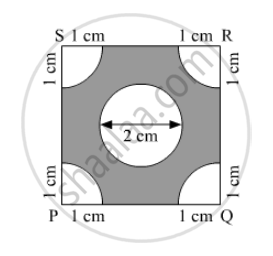Exercise 13.4 | Q 6

Four cows are tethered at four corners of a square plot of side 50m, so that’ they just cant reach one another. What area will be left ungrazed.

Exercise 13.4 | Q 7 | Page 56

A cow is tied with a rope of length 14 m at the corner of a rectangular  field of dimensions 20 m xx 16 m  find the area of the field in which the cow can graze .

Exercise 13.4 | Q 8 | Page 56

A calf is tied with a rope of length 6 m at the corner of a square grassy lawn of side 20 m . If the length of the rope is increased by 5.5 m , find the increase in area of the grassy lawn in which the calf can graze .

Exercise 13.4 | Q 9 | Page 56

A square water tank has its side equal to 40 m. There are four semi-circular grassy plots all round it. Find the cost of turfing the plot at Rs. 1.25 per square metre (Take π = 3.14).

Exercise 13.4 | Q 10 | Page 56

A rectangular park is 100 m by 50 m. It is surrounding by semi-circular flower beds all round. Find the cost of levelling the semi-circular flower beds at 60 paise per square metre (use π = 3.14).

Exercise 13.4 | Q 11 | Page 57

The inside perimeter of a running track (shown in the following figure) is 400 m. The length of each of the straight portion is 90 m and the ends are semi-circles. If the track is everywhere 14 m wide. find the area of the track. Also find the length of the outer running track.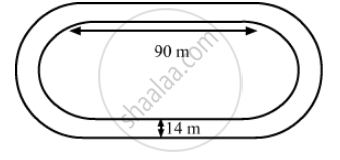Exercise 13.4 | Q 12 | Page 57

Find the area of the following figure, in square cm, correct to one place of decimal.  (Take π = 22/7).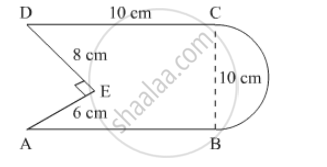Exercise 13.4 | Q 13 | Page 57

In the following figure, from a rectangular region ABCD with AB = 20 cm, a right triangle AED with AE = 9 cm and DE = 12 cm, is cut off. On the other end, taking BC as diameter, a semicircle is added on outside' the region. Find the area of the shaded region. [Use π =]22/7 [CBSE 2014]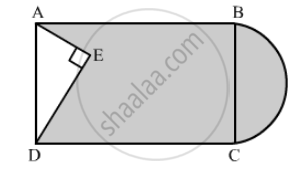Exercise 13.4 | Q 14 | Page 57

From each of the two opposite corners of a square of side 8 cm, a quadrant of a circle of radius 1.4 cm is cut. Another circle of radius 4.2 cm is also cut from the centre as shown in the following figure. Find the area of the remaining (Shaded) portion of the square. (Use π = 22/7)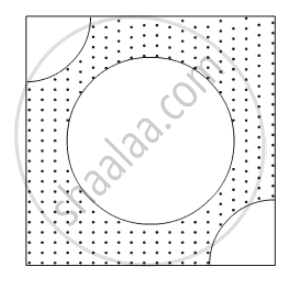Exercise 13.4 | Q 15

In the following figure, ABCD is a rectangle with AB = 14 cm and BC = 7 cm. Taking DCBC and AD as diameters, three semi-circles are drawn as shown in the figure. Find the area of the shaded region.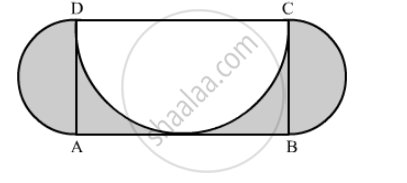Exercise 13.4 | Q 16.1 | Page 58

In the following figure, ABCD is a rectangle, having AB = 20 cm and BC = 14 cm. Two sectors of 180° have been cut off. Calculate:

the area of the shaded region.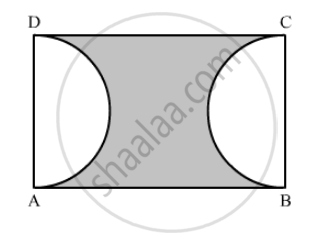Exercise 13.4 | Q 16.2

In the following figure, ABCD is a rectangle, having AB = 20 cm and BC = 14 cm. Two sectors of 180° have been cut off. Calculate:

the  length of the boundary of the shaded region.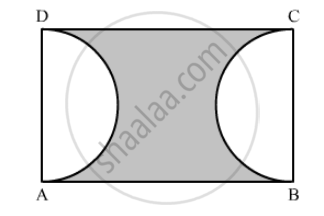Exercise 13.4 | Q 17.1 | Page 58

In the following figure, the square ABCD is divided into five equal parts, all having same area. The central part is circular and the ines AEGCBF and HD lie along the diagonals AC and BD of the square. If AB = 22 cm, find:

the circumference of the central part.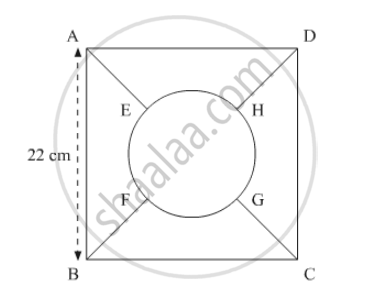Exercise 13.4 | Q 17.2 | Page 58

In the following figure, the square ABCD is divided into five equal parts, all having same area. The central part is circular and the lines AEGCBF and HD lie along the diagonals AC and BD of the square. If AB = 22 cm, find:

the perimeter of the part ABEF.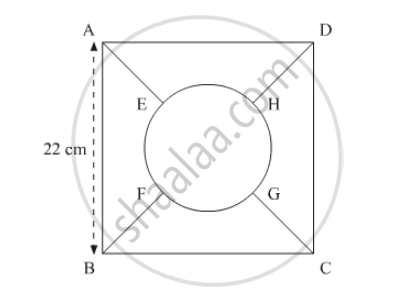Exercise 13.4 | Q 18 | Page 58

In the following figure find the area of the shaded region. (Use π = 3.14)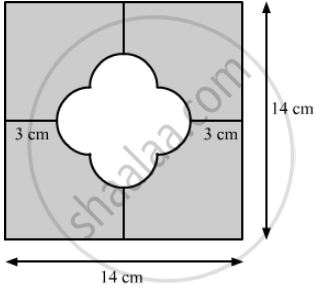Exercise 13.4 | Q 19 | Page 58

In the following figure, OACB is a quadrant of a circle with centre O and radius 3.5 cm. If OD = 2 cm, find the area of the (i) quadrant OACB (ii) shaded region.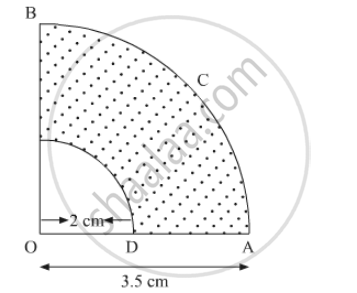Exercise 13.4 | Q 20 | Page 58

In the following figure a square OABC is inscribed in a quadrant OPBQ of a circle. If OA = 21 cm, find the area of the shaded region.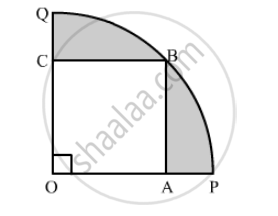Exercise 13.4 | Q 21 | Page 59

In the following figure, OABC is a square of side 7 cm. If OAPC is a quadrant of a circle with centre O, then find the area of the shaded region. (Use π = 22/7)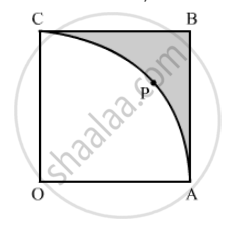Exercise 13.4 | Q 22 | Page 59

In the following figure, OE = 20 cm. In sector OSFT, square OEFG is inscribed. Find the area  of the shaded region.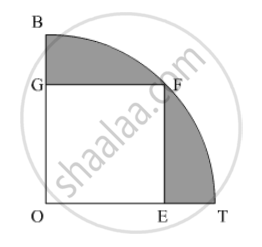Exercise 13.4 | Q 23 | Page 59

Find the area of the shaded region in the following figure, if AC = 24 cm, BC = 10 cm and O is the centre of the circle. (Use π = 3.14)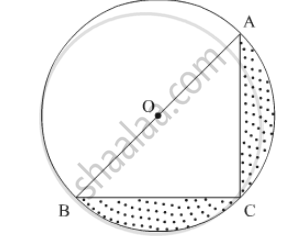Exercise 13.4 | Q 24 | Page 59

A circle is inscribed in an equilateral triangle ABC is side 12 cm, touching its sides (the following figure). Find the radius of the inscribed circle and the area of the shaded part.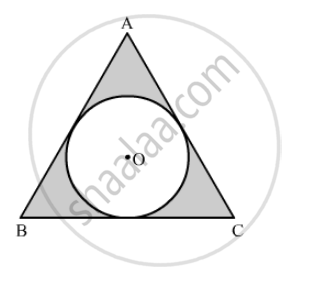Exercise 13.4 | Q 25 | Page 59

In the following figure, an equilateral triangle ABC of side 6 cm has been inscribed in a circle. Find the area of the shaded region. (Take π = 3.14).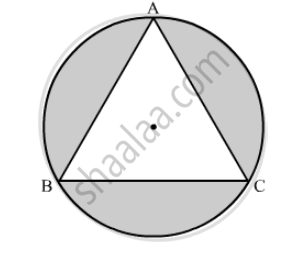Exercise 13.4 | Q 26 | Page 59

A circular field has a perimeter of 650 m. A square plot having its vertices on the circumference of the field is marked in the field. Calculate the area of the square plot.

Exercise 13.4 | Q 27 | Page 59

Find the area of a shaded region in the the following figure,where a circular arc of radius 7 cm has been drawn with vertex A of an equilateral triangle ABC of side 14 cm as centre.   (Use π = 22/7 and $\sqrt{3}$ = 1.73)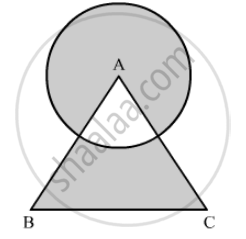Exercise 13.4 | Q 28 | Page 60

A regular hexagon is inscribed in a circle. If the area of hexagon is $24\sqrt{3}$ , find the area of the circle. (Use π = 3.14)

Exercise 13.4 | Q 29 | Page 60

ABCDEF is a regular hexagon with centre O (in the following figure). If the area of triangle OAB is 9 cm2, find the area of : (i) the hexagon and (ii) the circle in which the haxagon is incribed.

Exercise 13.4 | Q 30 | Page 60

Four equal circles, each of radius 5 cm touch each other as shown in fig. Find the area included etween them.

Exercise 13.4 | Q 31 | Page 60

Four equal circles each of radius a, touch each other. Show that area between them is 6/7a^2

Exercise 13.4 | Q 32 | Page 60

A child makes a poster on a chart paper drawing a square ABCD of side 14 cm. She draws four circles with centre A, B, C and D in which she suggests different ways to save energy. The circles are drawn in such a way that each circle touches externally two of the three remaining circles (in the following figure). In the shaded region she write a message 'Save Energy'. Find the perimeter and area of the shaded region.
(Use π = 22/7)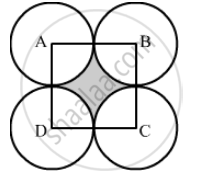Exercise 13.4 | Q 33 | Page 61

The diameter of a coin is 1 cm (in the following figure). If four such coins be placed on a table so that the rim of each touches that of the other two, find the area of the shaded region (Take π = 3.1416).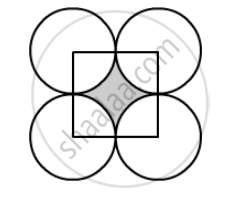Exercise 13.4 | Q 34 | Page 61

Two circular pieces of equal radii and maximum area, touching each other are cut out from a rectangular card board of dimensions 14 cm × 7 cm. Find the area of the remaining card board. (Use π = 22/7).

Exercise 13.4 | Q 34 | Page 61

Two circular pieces of equal radii and maximum area, touching each other are cut out from a rectangular card board of dimensions 14 cm × 7 cm. Find the area of the remaining card board. (Use π = 22/7).

Exercise 13.4 | Q 35 | Page 61

In the following figure, AB and CD are two diameters of a circle perpendicular to each other and OD is the diameter of the smaller circle. If OA = 7 cm, find the area of the shaded region.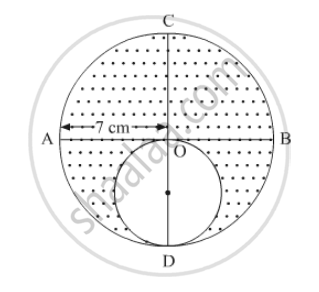Exercise 13.4 | Q 36 | Page 61

In the the following figure, PSR, RTQ and PAQ are three semicircles of diameter 10 cm, 3 cm and 7 cm respectively. Find the perimeter of shaded region.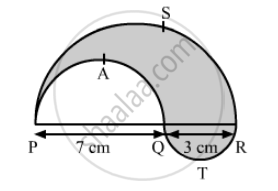Exercise 13.4 | Q 37 | Page 61

In the following figure, two circles with centres A and B touch each other at the point C. If AC = 8 cm and AB = 3 cm, find the area of the shaded region.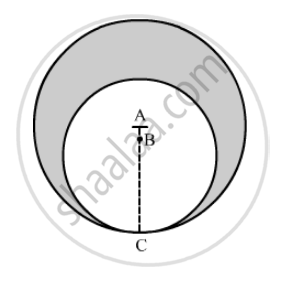Exercise 13.4 | Q 38 | Page 62

In the following figure, ABCD is a square of side 2a, Find the ratio between

(i) the circumferences

(ii) the areas of the in circle and the circum-circle of the square.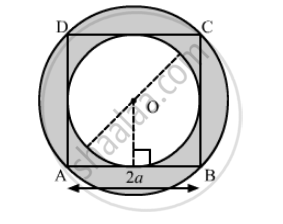Exercise 13.4 | Q 39 | Page 62

In the following figure, there are three semicircles, A, B and C having diameter 3 cm each, and another semicircle E having a circle D with diameter 4.5 cm are shown. Calculate:

(i) the area of the shaded region

(ii)  the cost of painting the shaded region at the rate of 25 paise per cm2 , to the nearest rupee.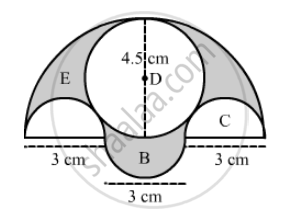Exercise 13.4 | Q 40 | Page 62

In the following figure, ABC is a right-angled triangle, ∠B = 90°, AB = 28 cm and BC = 21 cm. With AC as diameter a semicircle is drawn and with BC as radius a quarter circle is drawn. Find the area of the shaded region correct to two decimal places.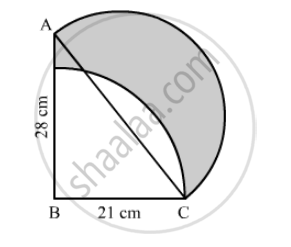Exercise 13.4 | Q 41 | Page 63

In the following figure, O is the centre of a circular arc and AOB is a straight line. Find the perimeter and the area of the shaded region correct to one decimal place. (Take π = 3.142)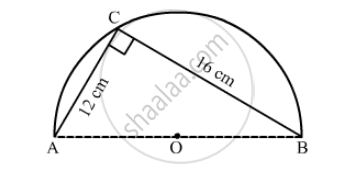Exercise 13.4 | Q 42 | Page 63

In the following figure, the boundary of the shaded region consists of four semi-circular arcs, the smallest two being equal. If the diameter of the largest is 14 cm and of the smallest is 3.5 cm, find

the length of the boundary.
the area of the shaded region.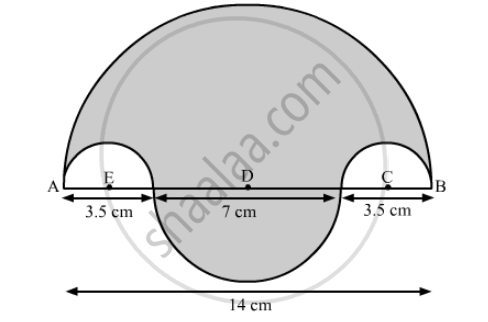Exercise 13.4 | Q 43 | Page 63

In the following figure, AB = 36 cm and M is mid-point of AB. Semi-circles are drawn on ABAM and MB as diameters. A circle with centre C touches all the three circles. Find the area of the shaded region.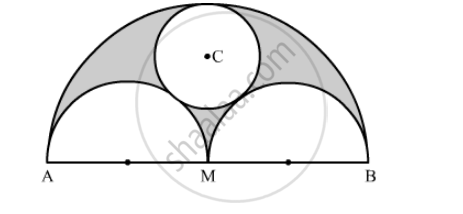Exercise 13.4 | Q 44 | Page 63

In the following figure, ABC is a right angled triangle in which ∠A = 90°, AB = 21 cm and AC = 28 cm. Semi-circles are described on ABBC and AC as diameters. Find the area of the shaded region.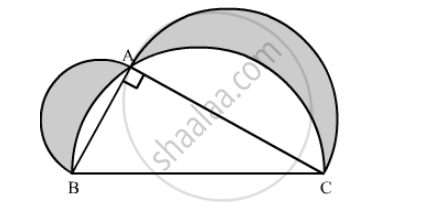Exercise 13.4 | Q 45.1 | Page 64

In the following figure, shows the cross-section of railway tunnel. The radius OA of the circular part is 2 m. If ∠AOB = 90°, calculate:
the height of the tunnel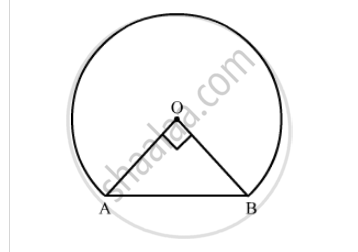Exercise 13.4 | Q 45.2 | Page 64

In the following figure, shows the cross-section of railway tunnel. The radius OA of the circular part is 2 m. If ∠AOB = 90°, calculate:

the perimeter of the cross-section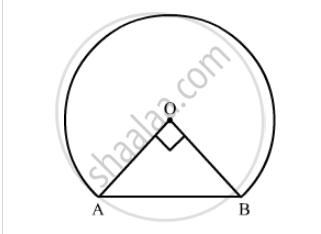Exercise 13.4 | Q 45.3 | Page 63

In the following figure, shows the cross-section of railway tunnel. The radius OA of the circular part is 2 m. If ∠AOB = 90°, calculate:

the area of the cross-section.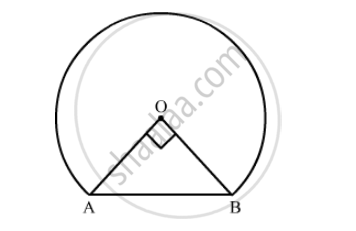Exercise 13.4 | Q 46 | Page 64

In the following figure, shows a kite in which BCD is the shape of a quadrant of a circle of radius 42 cm. ABCD is a square and Δ CEF is an isosceles right angled triangle whose equal sides are 6 cm long. Find the area of the shaded region.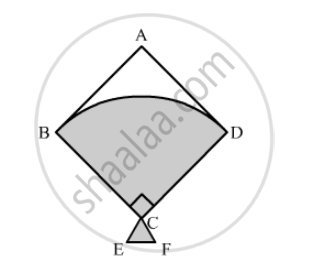Exercise 13.4 | Q 47 | Page 64

In the following figure, ABCD is a trapezium of area 24.5 cm2 , If AD || BC, ∠DAB = 90°, AD = 10 cm, BC = 4 cm and ABE is quadrant of a circle, then find the area of the shaded region. [CBSE 2014]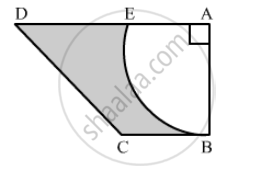Exercise 13.4 | Q 48 | Page 64

In the given figure, ABCD is a trapezium with AB || DC, AB = 18 cm DC = 32 cm and the distance between AB and DC is 14 cm. Circles of equal radii 7 cm with centres A, B, C and D have been drawn. Then find the area of the shaded region.
(Use $\pi = \frac{22}{7}$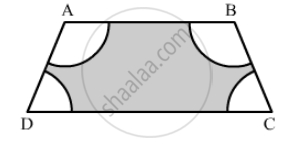Exercise 13.4 | Q 49 | Page 64

From a thin metallic piece, in the shape of a trapezium ABCD, in which AB || CD and ∠BCD = 90°, a quarter circle BEFC is removed (in the following figure). Given AB = BC = 3.5 cm and DE = 2 cm, calculate the area of the remaining piece of the metal sheet.

Exercise 13.4 | Q 50 | Page 65

In the following figure, ABC is an equilateral triangle of side 8 cm. A, B and C are the centres of circular arcs of radius 4 cm. Find the area of the shaded region correct upto 2 decimal places. (Take π =3.142 andsqrt3 = 1.732).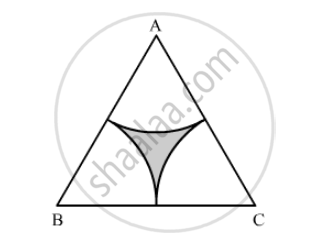Exercise 13.4 | Q 51 | Page 65

Sides of a triangular field are 15 m , 16 m , 17 m . With three corners of the field a cow , a buffalo and a horse are tied separately with ropes of length 7 m each to graze in the field . Find the area of the field which cannot be grazed by three  animals.

Exercise 13.4 | Q 52 | Page 65

In the given figure, the side of square is 28 cm and radius of each circle is half of the length of the side of the square where O and O' are centres of the circles. Find the area of shaded region.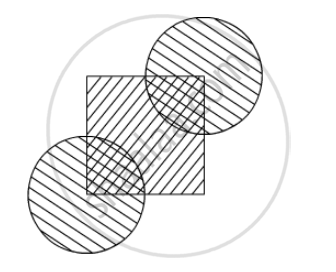Exercise 13.4 | Q 53 | Page 65

In a hospital used water is collected in a cylindrical tank of diameter 2 m and height 5 m. After recycling, this water is used to irrigate a park of hospital whose length is 25 m and breadth is 20 m. If tank is filled completely then what will be the height of standing water used for irrigating the park.

Exercise 13.5 [Pages 67 - 68]

### RD Sharma solutions for Class 10 Maths Chapter 13 Areas Related to Circles Exercise 13.5 [Pages 67 - 68]

Exercise 13.5 | Q 1 | Page 67

What is the ratio of the areas of a circle and an equilateral triangle whose diameter and a side are respectively equal?

Exercise 13.5 | Q 2 | Page 67

If the circumference of two circles are in the ratio  2 : 3, what is the ratio of their areas?

Exercise 13.5 | Q 3 | Page 67

Write the area of the sector of a circle whose radius is r and length of the arc is l.

Exercise 13.5 | Q 4 | Page 67

What is the length (in terms of π)  of the arc that subtends an angle of 36° at the centre of a circle of radius 5 cm?

Exercise 13.5 | Q 5 | Page 67

What is the angle subtended at the centre of a circle of radius 6 cm by an arc of length 3 π cm?

Exercise 13.5 | Q 6 | Page 68

What is the area of a sector of a circle of radius 5 cm formed by an arc of length 3.5 cm?

Exercise 13.5 | Q 7 | Page 68

In a circle of radius 10 cm, an arc subtends an angle of 108° at the centre. what is the area of the sector in terms of π?

Exercise 13.5 | Q 8 | Page 68

If a square is inscribed in a circle, what is the ratio of the areas of the circle and the square?

Exercise 13.5 | Q 9 | Page 68

Write the formula for the area of a sector of angle $\theta$ (in degrees) of a circle of radius r.

Exercise 13.5 | Q 10 | Page 68

Write the formula for the area of a segment in a circle of radius r given that the sector angle is $\theta$ (in degrees).

Exercise 13.5 | Q 11 | Page 68

If the adjoining figure is a sector of a circle of radius 10.5 cm, what is the perimeter of the sector? (Take $\pi = 22/7$)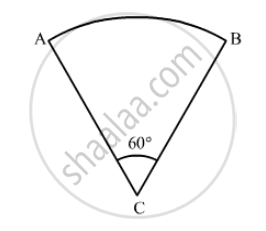Exercise 13.5 | Q 12 | Page 68

If the diameter of a semi-circular protractor is 14 cm, then find its perimeter.

Exercise 13.5 | Q 13 | Page 68

An arc subtends an angle of 90° at the centre of the circle of the radius 14 cm. Write the area of minor sector thus formed in terms of π.

Exercise 13.5 | Q 14 | Page 68

Find the area of the largest triangle that can be inscribed in a semi-circle of radius runits.

Exercise 13.5 | Q 15 | Page 68

Find the area of sector of circle  of radius 21 cm and central angle 1200.

Exercise 13.5 | Q 16 | Page 68

What is the area of a square inscribed in a circle  of diameter cm ?

Exercise 13.5 | Q 18 | Page 68

If the numerical value of the area of a circle is equal to the numerical value of its circumference , find its radius.

Exercise 13.5 | Q 19 | Page 68

How many revolutions a circular wheel of radius metres makes in covering a distance of s metres?

Exercise 13.5 | Q 20 | Page 68

Find the ratio of the area of the circle circumscribing a square to the area of the circle inscribed in the square .

Exercise 13.6 [Pages 69 - 74]

### RD Sharma solutions for Class 10 Maths Chapter 13 Areas Related to Circles Exercise 13.6 [Pages 69 - 74]

Exercise 13.6 | Q 1 | Page 69

If the circumference and the area of a circle are numerically equal, then diameter of the circle is

• $\frac{\pi}{2}$

•  2$\frac{\pi}{2}$

•  2

• 4

Exercise 13.6 | Q 2 | Page 69

If the difference between the circumference and radius of a circle is 37 cm, then using π = $\frac{22}{7}$ the circumference (in cm) of the circle is

•  154

•  44

• 14

• 7

Exercise 13.6 | Q 3 | Page 69

A wire can be bent in the form of a circle of radius 56 cm. If it is bent in the form fo a square, then its area will be

• 3520 cm2

• 6400 cm2

• 7744 cm2

• 8800 cm2

Exercise 13.6 | Q 4 | Page 69

If a wire is bent into the shape of a square, then the area of the square is 81 cm2 . When wire is bent into a semi-circular shape, then the  area of the semi-circle will be

• 22 cm2

• 44 cm2

• 77 cm2

•  154 cm2

Exercise 13.6 | Q 5 | Page 69

A circular park has a path of uniform width around it. The difference between the outer and inner circumferences of the circular path is 132 m. Its width is

• 20 m

•  21 m

• 22 m

• 24 m

Exercise 13.6 | Q 6 | Page 69

The radius of a wheel is 0.25 m. The number of revolutions it will make to travel a distance of 11 km will be

•  2800

• 4000

•  5500

•  7000

Exercise 13.6 | Q 7 | Page 69

The ratio of the outer and inner perimeters of a circular path is 23 : 22. If the path is 5 metres wide, the diameter of the inner circle is

• 55 m

•  110 m

• 220 m

• 230 m

Exercise 13.6 | Q 8 | Page 69

The circumference of a circle is 100 m. The side of a square inscribed in the circle is

•  50$\sqrt{2}$

• $\frac{50}{\pi}$

• $\frac{50\sqrt{2}}{\pi}$

• $\frac{100\sqrt{2}}{\pi}$

Exercise 13.6 | Q 9 | Page 69

The area of the incircle of an equilateral triangle of side 42 cm is

• $22\sqrt{3} c m^2$

•  231 cm2

• 462 cm2

• 924 cm2

Exercise 13.6 | Q 10 | Page 69

The area of incircle of an equilateral triangle is 154 cm2 . The perimeter of the triangle is

• 71.5 cm

• 71.7 cm

•  72.3 cm

• 72.7 cm

Exercise 13.6 | Q 11 | Page 69

The area of the largest triangle that can be inscribed in a semi-circle of radius r, is

• r2

•  2r2

• r3

• 2r3

Exercise 13.6 | Q 12 | Page 69

The perimeter of a triangle is 30 cm and the circumference of its incircle is 88 cm. The area of the triangle is

• 70 cm2

• 140 cm2

•  210 cm2

• 420 cm2

Exercise 13.6 | Q 13 | Page 69

The area of a circle is 220 cm2. The area of ta square inscribed in it is

• 49 cm2

• 70 cm2

•  140 cm2

•  150 cm

Exercise 13.6 | Q 14 | Page 70

If the circumference of a circle increases from 4π to 8π, then its area is

• halved

• doubled

• tripled

Exercise 13.6 | Q 15 | Page 70

If the radius of a circle is diminished by 10%, then its area is diminished by

• 10%

• 19%

• 20%

• 36%

Exercise 13.6 | Q 16 | Page 70

If the area of a square is same as the area of a circle, then the ratio of their perimeters, in terms of π, is

•  π :$\sqrt{3}$

• 2 : $\sqrt{\pi}$

•  3 :$\pi$

• $\pi : \sqrt{2}$

Exercise 13.6 | Q 17 | Page 70

The area of the largest triangle that can be inscribed in a semi-circle of radius r is

• 2r

•  r2

• r

• $\sqrt{r}$

Exercise 13.6 | Q 18 | Page 70

The ratio of the areas of a circle and an equilateral triangle whose diameter and a side are respectively equal, is

• $\pi: \sqrt{2}$

• $\pi: \sqrt{3}$

• $\sqrt{3}: \pi$

• $\sqrt{2}: \pi$

Exercise 13.6 | Q 19 | Page 70

If the sum of the areas of two circles with radii r1 and r2 is equal to the area of a circle of radius r, then  $r_1^2 + r_2^2$

•  >r2

• =r2

• <r2

•  None of these

Exercise 13.6 | Q 20 | Page 70

If the perimeter of a semi-circular protractor is 36 cm, then its diameter is

• 10 cm

• 12 cm

• 14 cm

• 16 cm

Exercise 13.6 | Q 21 | Page 70

The perimeter of the sector OAB shown in the following figure,  is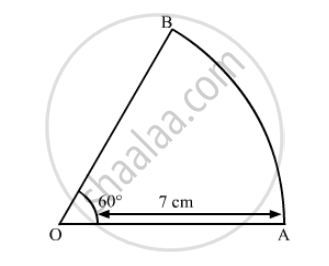• $\frac{64}{3} cm$

• 26 cm

• $\frac{64}{5} cm$

•  19 cm

Exercise 13.6 | Q 22 | Page 70

If the perimeter of a sector of a circle of radius 6.5 cm is 29 cm, then its area is

•  58 cm2

• 52 cm2

•  25 cm2

• 56 cm2

Exercise 13.6 | Q 23 | Page 70

If the area of a sector of a circle bounded by an arc of length 5π cm is equal to 20π cm2, then its radius is

• 12 cm

• 16 cm

• 8 cm

•  10 cm

Exercise 13.6 | Q 24 | Page 70

The area of the circle that can be inscribed in a square of side 10 cm is

• 40 π cm2

• 30 π cm2

•  100 π cm2

• 25 π cm2

Exercise 13.6 | Q 25 | Page 71

If the difference between the circumference and radius of a circle is 37 cm, then its area is

• 154 cm2

• 160 cm2

•  200 cm2

• 150 cm2

Exercise 13.6 | Q 26 | Page 71

The area of a circular path of uniform width h surrounding a circular region of radius r is

• $\pi(2r + h)r$

• $\pi(2r + h)r$

• $\pi(2r + h)h$

• $\pi(h + r)r$

• $\pi(h + r)h$

Exercise 13.6 | Q 27 | Page 71

If AB is a chord of length $5\sqrt{3}$ cm of a circle with centre O and radius 5 cm, then area of sector OAB is

• $\frac{3\pi}{8}c m^2$

• $\frac{8\pi}{3}c m^2$

• $25 \pi cm^2$

• $\frac{25\pi}{3}c m^2$

Exercise 13.6 | Q 28 | Page 71

The area of a circle whose area and circumference are numerically equal, is

• 2 $\pi$ sq. units

• 4 $\pi$ units

• 6$\pi$sq. units

• 8 $\pi$ sq. units

Exercise 13.6 | Q 29 | Page 71

If diameter of a circle is increased by 40%, then its area increase by

• 96%

• 40%

• 80%

•  48%

Exercise 13.6 | Q 30 | Page 71

In the following figure, the shaded area is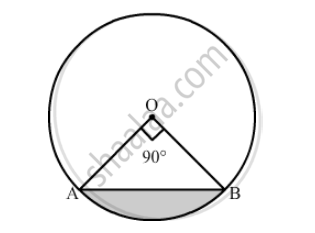•  50 (π−2) cm2

•  25 (π−2) cm2

•  25 (π+2) cm2

•  5 (π−2) cm2

Exercise 13.6 | Q 31 | Page 71

In the following figure, the area of the segment PAQ is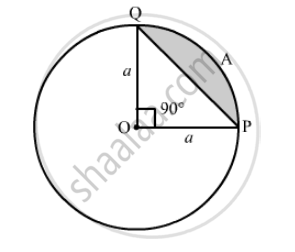• $\frac{a^2}{4}\left( \pi + 2 \right)$

• $\frac{a^2}{4}\left( \pi - 2 \right)$

• $\frac{a^2}{4}\left( \pi - 1 \right)$

• $\frac{a^2}{4}\left( \pi + 1 \right)$

Exercise 13.6 | Q 33 | Page 71

If the area of a sector of a circle bounded by an arc of length 5π  cm is equal to 20π cm2, then the radius of the circle

• 12 cm

• 16 cm

• 8 cm

•  10 cm

Exercise 13.6 | Q 35 | Page 71

If the area of a sector of a circle is 5/18 of the area of the circle, then the sector angle is equal to

• 60°

• 90°

• 100°

• 120°

Exercise 13.6 | Q 36 | Page 72

If he area of a sector of a circle is $\frac{7}{20}$ of the area of the circle, then the sector angle is equal to

• 110°

• 130°

• 100°

• 126°

Exercise 13.6 | Q 37 | Page 72

In the following figure, If ABC is an equilateral triangle, then shaded area is equal to

• $\left( \frac{\pi}{3} - \frac{\sqrt{3}}{4} \right) r^2$

• $\left( \frac{\pi}{3} - \frac{\sqrt{3}}{2} \right) r^2$

• $\left( \frac{\pi}{3} + \frac{\sqrt{3}}{4} \right) r^2$

• $\left( \frac{\pi}{3} + \sqrt{3} \right) r^2$

Exercise 13.6 | Q 38 | Page 73

In the following figure, the area of the shaded region is

•  3π cm2

• 6π cm2

• 9π cm2

• 7π cm2

Exercise 13.6 | Q 39 | Page 73

If the perimeter of a circle is equal to that of a square, then the ratio of their areas is ______.

•  13 : 22

• 14 : 11

•  22 : 13

• 11 : 14

Exercise 13.6 | Q 40 | Page 73

The radius of a circle is 20 cm. It is divided into four parts of equal area by drawing three concentric circles inside it. Then, the radius of the largest of three concentric circles drawn is

• 10 $\sqrt{5}$

• 10$\sqrt{3}$cm

• 10$\sqrt{5}$

•  10$\sqrt{2}$

Exercise 13.6 | Q 41 | Page 73

The area of a sector whose perimeter is four times its radius r units, is

• $\frac{r^2}{4}$

• 2r2  sq. units

•  r2 sq.units

Exercise 13.6 | Q 42 | Page 73

If a chord of a circle of  radius 28 cm makes an angle of 90 ° at the centre, then the area of the major segment is

• 392 cm2

•  1456 cm2

• 1848 cm2

• 2240 cm2

Exercise 13.6 | Q 43 | Page 73

If area of a circle inscribed in an equilateral triangle is 48π square units, then perimeter of the triangle is

•  17 $\sqrt{3}$units

• 36 units

• 72 units

• 48$\sqrt{3}$units

Exercise 13.6 | Q 44 | Page 74

The hour hand of a clock is 6 cm long. The area swept by it between 11.20 am and 11.55 am is

• 2.75 cm2

• 5.5 cm

• 11 cm2

• 10 cm2

Exercise 13.6 | Q 45 | Page 74

ABCD is a square of side 4 cm. If E is a point in the interior of the square such that ΔCEDis equilateral, then area of Δ ACE is

• $2\sqrt{3} - 1 c m^2$

• $4\sqrt{3} - 1 c m^2$

• $6\sqrt{3} - 1 c m^2$

• $8\sqrt{3} - 1 c m^2$

Exercise 13.6 | Q 46 | Page 74

If the area of a circle is equal to the sum of the areas of two circles of diameters 10 cm and 24 cm, then diameter of the large circle (in cm) is

• 34

• 26

•  17

• 14

Exercise 13.6 | Q 47 | Page 74

If π is taken as 22/7 the distance (in metres) covered by a wheel of diameter 35 cm, in one revolution, is

•  2.2

• 1.1

•  9.625

•  96.25

Exercise 13.6 | Q 48 | Page 74

ABCD is a rectangle whose three vertices are B (4,0), C (4,3) and D (0, 3). The length of one of its diagonals is

•  5

• 4

• 3

• 25

Exercise 13.6 | Q 49 | Page 74

Area of the largest triangle that can be inscribed in a semi-circle of radius r units is ______.

• rsq.units

• 1/2 rsq.units

•  2 rsq.units

• sqrt(2) rsq.units

Exercise 13.6 | Q 50 | Page 74

If the sum of the areas of two circles with radii $r_1$and $r_2$ is equal to the area of circle of radius $r$ then

• $r = r_1 + r_2$

• ${r_1}^2 + {r_2}^2 = r^2$

• $r_1 + r_2 < r$

• ${r_1}^2 + {r_2}^2 < r^2$

Exercise 13.6 | Q 51 | Page 74

If the sum of the circumferences of the two circles with radii $r_1$ and  $r_2$ is equal to the circumference of a circle of radius $r$ then

• $r = r_1 + r_2$

• $r_1 + r_2 > r$

• $r_1 + r_2 < r$

• None of these

Exercise 13.6 | Q 52 | Page 74

If the circumference of a circle and the perimeter of a square are equal, then ______.

• Area of the circle = Area of the square

• Area of the circle  < Area of the  square

• Area of the circle > Area of the square

• Nothing definite can be said about the relation between the areas of the circle and square.

Exercise 13.6 | Q 53 | Page 74

If the perimeter of a circle is equal to that of a square, then the ratio of their areas is ______.

• 22 : 7

• 14 : 11

•  7 : 22

•  11 : 14

## Chapter 13: Areas Related to Circles

Exercise 13.1Exercise 13.2Exercise 13.3Exercise 13.4Exercise 13.5Exercise 13.6## RD Sharma solutions for Class 10 Maths chapter 13 - Areas Related to Circles

RD Sharma solutions for Class 10 Maths chapter 13 (Areas Related to Circles) include all questions with solution and detail explanation. This will clear students doubts about any question and improve application skills while preparing for board exams. The detailed, step-by-step solutions will help you understand the concepts better and clear your confusions, if any. Shaalaa.com has the CBSE Class 10 Maths solutions in a manner that help students grasp basic concepts better and faster.

Further, we at Shaalaa.com provide such solutions so that students can prepare for written exams. RD Sharma textbook solutions can be a core help for self-study and acts as a perfect self-help guidance for students.

Concepts covered in Class 10 Maths chapter 13 Areas Related to Circles are Areas of Sector and Segment of a Circle, Area of Circle, Areas of Combinations of Plane Figures, Circumference of a Circle, Circumference of a Circle, Circles Passing Through One, Two, Three Points, Converse of Tangent Theorem, Tangent Segment Theorem, Inscribed Angle Theorem, Corollaries of Inscribed Angle Theorem, Theorem: Opposite angles of a cyclic quadrilateral are supplementary., Corollary of Cyclic Quadrilateral Theorem, Converse: If a Pair of Opposite Angles of a Quadrilateral is Supplementary, Then the Quadrilateral is Cyclic., Theorem of Angle Between Tangent and Secant, Converse of Theorem of the Angle Between Tangent and Secant, Theorem of Internal Division of Chords, Theorem of External Division of Chords, Tangent to a Circle, Number of Tangents from a Point on a Circle, Touching Circles, Concept of Circle - Centre, Radius, Diameter, Arc, Sector, Chord, Segment, Semicircle, Circumference, Interior and Exterior, Concentric Circles, Secant and Tangent, Theorem of Touching Circles, Tangent Properties - If Two Circles Touch, the Point of Contact Lies on the Straight Line Joining Their Centers, Introduction to an Arc, Congruence of Arcs, Property of Sum of Measures of Arcs, Inscribed Angle, Intercepted Arc, Cyclic Quadrilateral, Converse of Cyclic Quadrilateral Theorem, Tangent Secant Segments Theorem, Tangent - Secant Theorem, Angle Subtended by the Arc to the Point on the Circle, Angle Subtended by the Arc to the Centre.

Using RD Sharma Class 10 solutions Areas Related to Circles exercise by students are an easy way to prepare for the exams, as they involve solutions arranged chapter-wise also page wise. The questions involved in RD Sharma Solutions are important questions that can be asked in the final exam. Maximum students of CBSE Class 10 prefer RD Sharma Textbook Solutions to score more in exam.

Get the free view of chapter 13 Areas Related to Circles Class 10 extra questions for Class 10 Maths and can use Shaalaa.com to keep it handy for your exam preparation